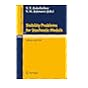Normal view

# Stability problems for stochastic models : proceedings of the 9th international seminar held in Varna, Bulgaria, May 13-19, 1985 / edited by V.V. Kalashnikov, B. Penkov, and V.M. Zolotarev.

Material type:TextSeries: Lecture notes in mathematics (Springer-Verlag): 1233.Publisher: Berlin ; New York : Springer-Verlag, ©1987Description: 1 online resource (vi, 223 pages)Content type: text Media type: computer Carrier type: online resourceISBN: 9783540473947; 3540473947Additional physical formats: Print version:: Stability problems for stochastic models.DDC classification: 510 s | 003 LOC classification: QA402 | .S73 1987ebQA3 | .L28 no. 1233 | QA402Online resources: Click here to access online
Contents:
The estimation of the rate of convergence in the integral limit theorem in the Euclidean motion group -- Contribution to the analytic theory of linear forms of independent random variables --?p-strictly stable laws and estimation of their parameters -- The method of metric distances in the problem of estimation of the deviation from the exponential distribution -- The accuracy of the normal approximation to the distribution of the sum of a random number of independent random variables -- Mixtures of probability distributions -- Some limit theorems for summability methods of I.I.D. Random variables -- Properties of mode of spectral positive stable distributions -- Two characterizations using records -- On orthogonal-series estimators for probability distributions -- Estimates of the deviation between the exponential and new classes of bivariate distributions -- On the difference between distributions of sums and maxima -- On the inequalities of Berry-Esseen and V.M. Zolotarev -- Some fixed point theorems probabilistic metric spaces -- The asymptotic bias in a deviation of a location model -- Cramer's decomposition theorem within the continuation of distribution functions -- An asymptotically most Bias-Robust invariant estimator of location -- Characterizing the distributions of the random vectors X 1, X 2, X 3 by the distribution of the statistic (X 1-X 3, X 2-X 3) -- On stability estimates of Cramer's theorem -- On the estimation of moments of regenerative cycles in a general closed central-server queueing network -- On F-processes and their applications -- On some properties of ideal metrics of order? -- On?-independence of sample mean and sample variance.
Action note: digitized 2011 committed to preserve
Tags from this library: No tags from this library for this title.Average rating: 0.0 (0 votes)
Item type Current location Collection Call number Status Date due Barcode Item holdseBook e-Library

Electronic Book@IST

EBook Available
Total holds: 0

Includes bibliographical references.

Print version record.

The estimation of the rate of convergence in the integral limit theorem in the Euclidean motion group -- Contribution to the analytic theory of linear forms of independent random variables --?p-strictly stable laws and estimation of their parameters -- The method of metric distances in the problem of estimation of the deviation from the exponential distribution -- The accuracy of the normal approximation to the distribution of the sum of a random number of independent random variables -- Mixtures of probability distributions -- Some limit theorems for summability methods of I.I.D. Random variables -- Properties of mode of spectral positive stable distributions -- Two characterizations using records -- On orthogonal-series estimators for probability distributions -- Estimates of the deviation between the exponential and new classes of bivariate distributions -- On the difference between distributions of sums and maxima -- On the inequalities of Berry-Esseen and V.M. Zolotarev -- Some fixed point theorems probabilistic metric spaces -- The asymptotic bias in a deviation of a location model -- Cramer's decomposition theorem within the continuation of distribution functions -- An asymptotically most Bias-Robust invariant estimator of location -- Characterizing the distributions of the random vectors X 1, X 2, X 3 by the distribution of the statistic (X 1-X 3, X 2-X 3) -- On stability estimates of Cramer's theorem -- On the estimation of moments of regenerative cycles in a general closed central-server queueing network -- On F-processes and their applications -- On some properties of ideal metrics of order? -- On?-independence of sample mean and sample variance.

Use copy Restrictions unspecified star MiAaHDL

Electronic reproduction. [Place of publication not identified] : HathiTrust Digital Library, 2011. MiAaHDL

Master and use copy. Digital master created according to Benchmark for Faithful Digital Reproductions of Monographs and Serials, Version 1. Digital Library Federation, December 2002. MiAaHDL

digitized 2011 HathiTrust Digital Library committed to preserve pda MiAaHDL

There are no comments for this item.Stability problems for stochastic models : ©1989Stability problems for stochastic models : ©1983Stability problems for stochastic models : ©1993Stability problems for stochastic models : ©1989Stability problems for stochastic models : ©1993Stability problems for stochastic models : ©1985Stability problems for stochastic models : ©1983